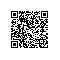# MATLAB把ISE中产生的1QN格式的十六进制数据转换为十进制数进行分析

N=64;
fid = fopen('e:\sin.txt','r');
for i = 1 : N;
num(i) = fscanf(fid, '%x', 1)   %从fid所指的文件以16进制方式读出数据
if(num(i)>512)
num(i)=-(num(i)-512)/2^8;%第10位（符号位）是1，转换为负数.8位小数位，除以2^8
else
num(i)=num(i)/2^8;
end
end
fclose(fid);
plot(num)使用钉钉扫一扫加入圈子
+ 订阅